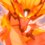# Fractional Part Integral Open Problem

Find a closed form of

$\int_{0}^{1} \ldots \int_{0}^{1} \left \{ \dfrac{x_{1}}{x_{2}} \right \} \left \{\dfrac{x_{2}}{x_{3}}\right \} \ldots \left \{\dfrac{x_{n-1}}{x_{n}}\right \} \left \{\dfrac{x_{n}}{x_{1}}\right \} \ \mathrm{d}x_{1} \ \mathrm{d}x_{2} \ldots \mathrm{d}x_{n} \quad ; \quad n \geq 3$

Notation : $\{ \cdot \}$ denotes fractional part function.

This is a part of the set Formidable Series and IntegralsNote by Ishan Singh
5 years, 2 months ago

This discussion board is a place to discuss our Daily Challenges and the math and science related to those challenges. Explanations are more than just a solution — they should explain the steps and thinking strategies that you used to obtain the solution. Comments should further the discussion of math and science.

When posting on Brilliant:

• Use the emojis to react to an explanation, whether you're congratulating a job well done , or just really confused .
• Ask specific questions about the challenge or the steps in somebody's explanation. Well-posed questions can add a lot to the discussion, but posting "I don't understand!" doesn't help anyone.
• Try to contribute something new to the discussion, whether it is an extension, generalization or other idea related to the challenge.
• Stay on topic — we're all here to learn more about math and science, not to hear about your favorite get-rich-quick scheme or current world events.

MarkdownAppears as
*italics* or _italics_ italics
**bold** or __bold__ bold
- bulleted- list
• bulleted
• list
1. numbered2. list
1. numbered
2. list
Note: you must add a full line of space before and after lists for them to show up correctly
paragraph 1paragraph 2

paragraph 1

paragraph 2

[example link](https://brilliant.org)example link
> This is a quote
This is a quote
    # I indented these lines
# 4 spaces, and now they show
# up as a code block.

print "hello world"
# I indented these lines
# 4 spaces, and now they show
# up as a code block.

print "hello world"
MathAppears as
Remember to wrap math in $$ ... $$ or $ ... $ to ensure proper formatting.
2 \times 3 $2 \times 3$
2^{34} $2^{34}$
a_{i-1} $a_{i-1}$
\frac{2}{3} $\frac{2}{3}$
\sqrt{2} $\sqrt{2}$
\sum_{i=1}^3 $\sum_{i=1}^3$
\sin \theta $\sin \theta$
\boxed{123} $\boxed{123}$

## Comments

Sort by:

Top Newest

Damn. Its looks so difficult :D it is easier to solve a rubic's cube in 1 min rather solving this problem :p

- 5 years, 2 months ago

Log in to reply

i was making good progress,but i got stuck at

$\displaystyle\int _{ 0 }^{ 1 }{ \left\lfloor \frac { { x }_{ 1 } }{ { x }_{ 2 } } \right\rfloor \left\lfloor \frac { { x }_{ 3 } }{ { x }_{ 1 } } \right\rfloor d{ x }_{ 1 } }$

:(

- 5 years, 2 months ago

Log in to reply

okay i will try

- 5 years, 1 month ago

Log in to reply

Case $n=2$ is very simple.

- 5 years, 2 months ago

Log in to reply

ya right :)

- 5 years, 2 months ago

Log in to reply

×

Problem Loading...

Note Loading...

Set Loading...